# Introduction to Sorting What is Sorting Sorting an

• Slides: 19
Download presentationIntroduction to Sorting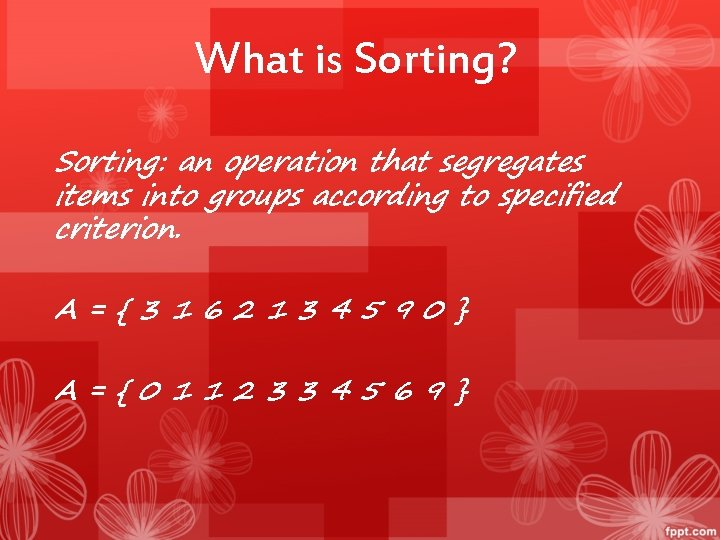What is Sorting? Sorting: an operation that segregates items into groups according to specified criterion. A={3162134590} A={0112334569}Why Sort and Examples Consider: • • Sorting Books in Library (Dewey system) Sorting Individuals by Height (Feet and Inches) Sorting Movies in Blockbuster (Alphabetical) Sorting Numbers (Sequential)Types of Sorting Algorithms There are many, many different types of sorting algorithms, but the primary ones are: ● ● ● Bubble Sort Selection Sort Insertion Sort Merge Sort Shell Sort Heap Sort ● ● ● Quick Sort Radix Sort Swap SortReview of Complexity Most of the primary sorting algorithms run on different space and time complexity. Time Complexity is defined to be the time the computer takes to run a program (or algorithm in our case). Space complexity is defined to be the amount of memory the computer needs to run a program.Complexity (cont. ) Complexity in general, measures the algorithms efficiency in internal factors such as the time needed to run an algorithm. External Factors (not related to complexity): • Size of the input of the algorithm • Speed of the Computer • Quality of the Compiler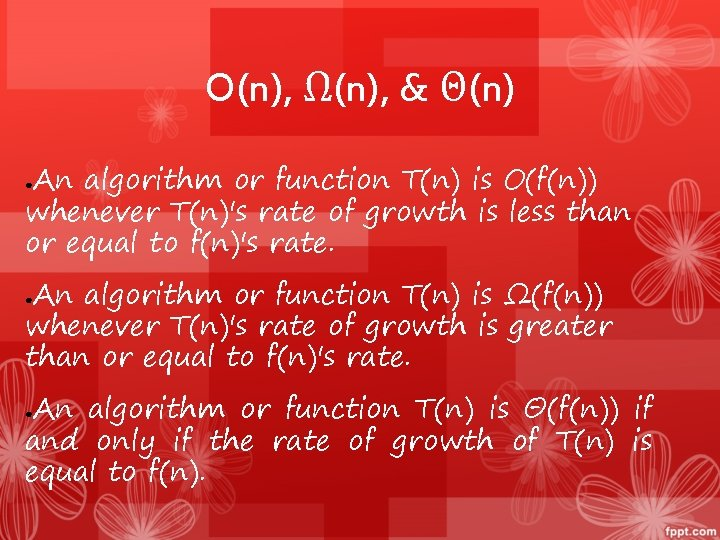O(n), Ω(n), & Θ(n) An algorithm or function T(n) is O(f(n)) whenever T(n)'s rate of growth is less than or equal to f(n)'s rate. ● An algorithm or function T(n) is Ω(f(n)) whenever T(n)'s rate of growth is greater than or equal to f(n)'s rate. ● An algorithm or function T(n) is Θ(f(n)) if and only if the rate of growth of T(n) is equal to f(n). ●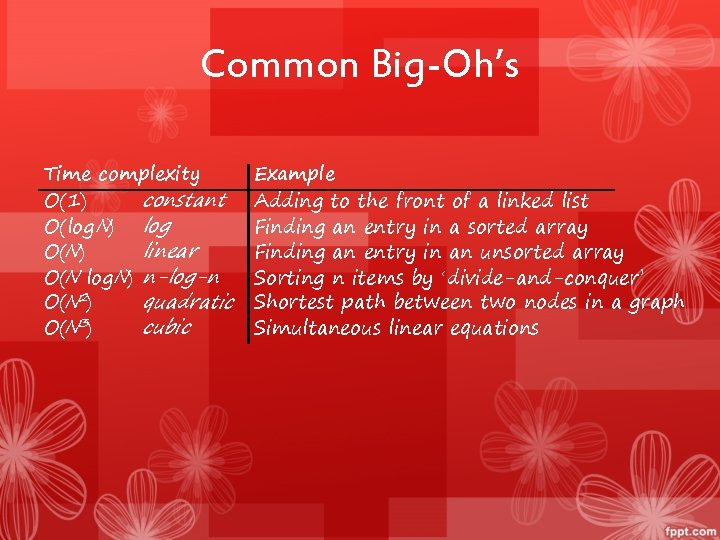Common Big-Oh’s Time complexity constant O(1) O(log N) log linear O(N) O(N log. N) n-log-n quadratic O(N 2) cubic O(N 3) Example Adding to the front of a linked list Finding an entry in a sorted array Finding an entry in an unsorted array Sorting n items by ‘divide-and-conquer’ Shortest path between two nodes in a graph Simultaneous linear equations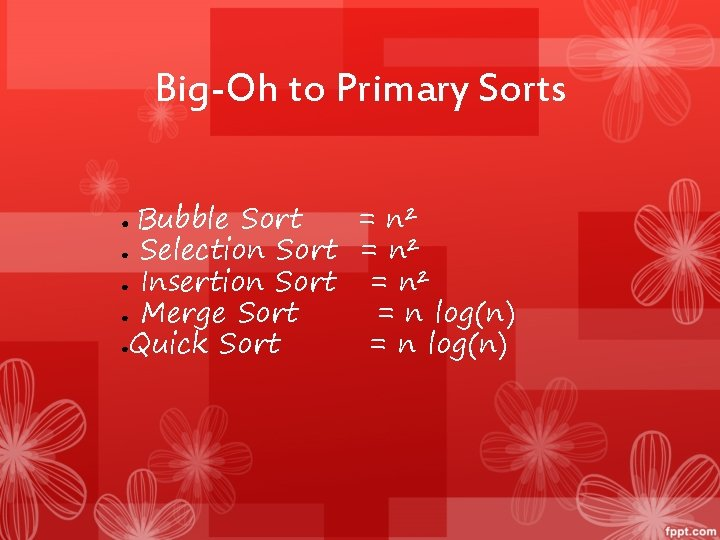Big-Oh to Primary Sorts Bubble Sort = n² ● Selection Sort = n² ● Insertion Sort = n² ● Merge Sort = n log(n) ●Quick Sort = n log(n) ●Time Efficiency • How do we improve the time efficiency of a program? • The 90/10 Rule 90% of the execution time of a program is spent in executing 10% of the code • So, how do we locate the critical 10%? • software metrics tools • global counters to locate bottlenecks (loop executions, function calls)Time Efficiency Improvements Possibilities (some better than others!) • Move code out of loops that does not belong there (just good programming!) • Remove any unnecessary I/O operations (I/O operations are expensive time-wise) • Code so that the compiled code is more efficient Moral - Choose the most appropriate algorithm(s) BEFORE program implementation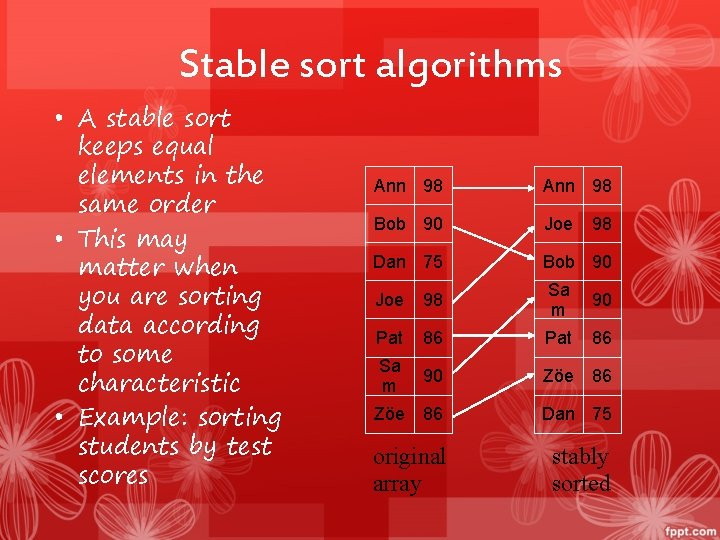Stable sort algorithms • A stable sort keeps equal elements in the same order • This may matter when you are sorting data according to some characteristic • Example: sorting students by test scores Ann 98 Bob 90 Joe Dan 75 Bob 90 Joe 98 Sa m 90 Pat 86 Sa m 90 Zöe 86 98 Zöe 86 Dan 75 original array stably sortedUnstable sort algorithms • An unstable sort may or may not keep equal elements in the same order • Stability is usually not important, but sometimes it is important Ann 98 Joe Bob 90 Ann 98 Dan 75 Bob 90 98 Joe 98 Sa m 90 Pat 86 Zöe 86 Dan 75 original array unstably sortedSelection Sorting Step: • 1. select the smallest element among data[i]~ data[data. length-1]; • 2. swap it with data[i]; • 3. if not finishing, repeat 1&2 20 8 5 10 7 5 8 20 10 7 5 7 20 10 8 5 7 8 10 20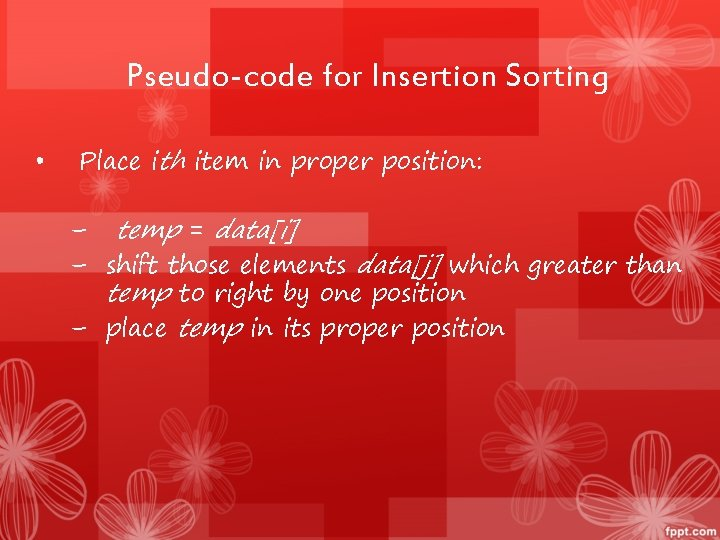Pseudo-code for Insertion Sorting • Place ith item in proper position: – temp = data[i] – shift those elements data[j] which greater than temp to right by one position – place temp in its proper positionInsert Action: i=1 temp 8 20 8 5 10 7 8 20 20 5 10 7 --- 8 20 5 10 7 i = 1, first iteration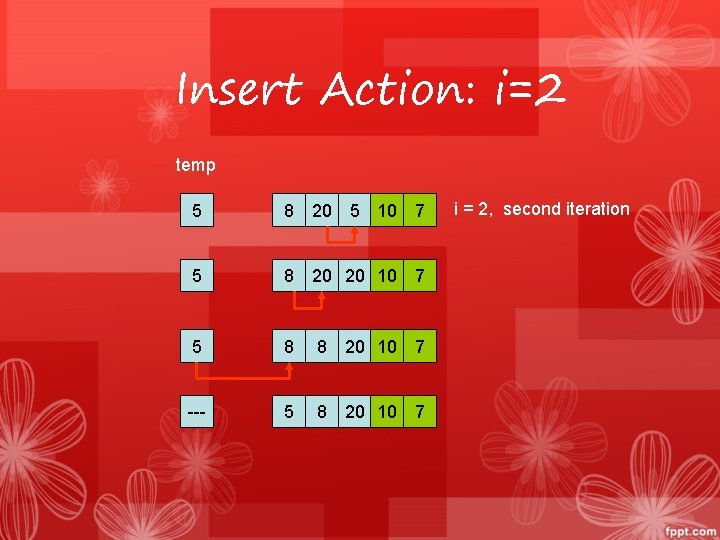Insert Action: i=2 temp 5 8 20 5 10 7 5 8 20 20 10 7 5 8 8 20 10 7 --- 5 8 20 10 7 i = 2, second iteration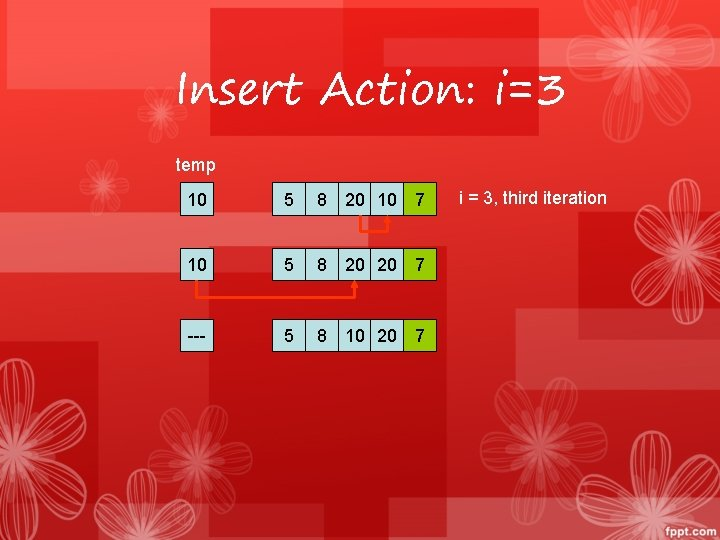Insert Action: i=3 temp 10 5 8 20 10 7 10 5 8 20 20 7 --- 5 8 10 20 7 i = 3, third iteration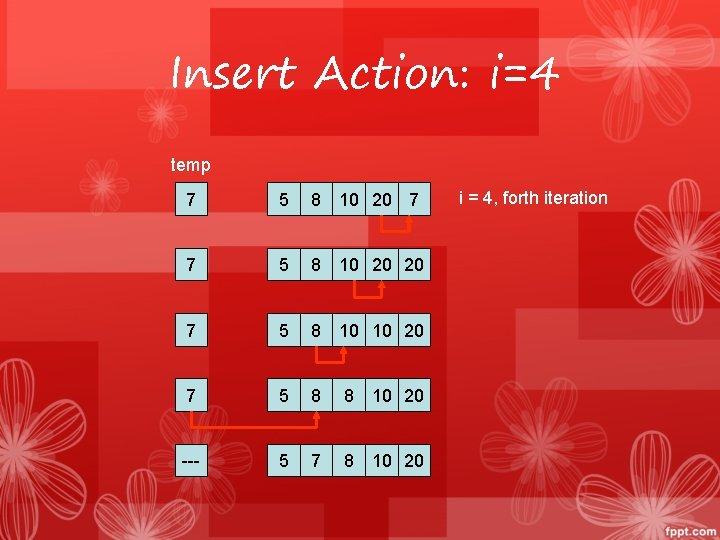Insert Action: i=4 temp 7 5 8 10 20 7 7 5 8 10 20 20 7 5 8 10 10 20 7 5 8 8 10 20 --- 5 7 8 10 20 i = 4, forth iteration